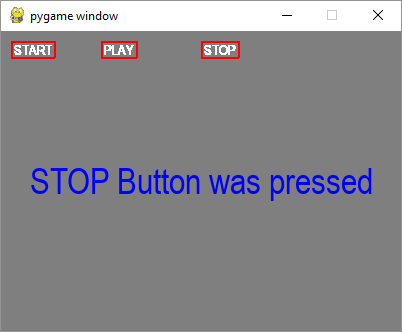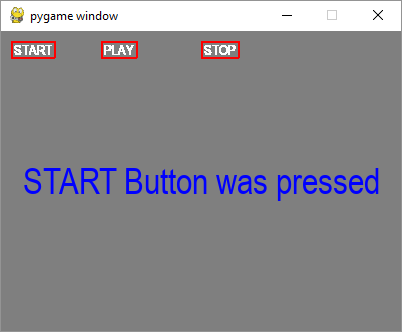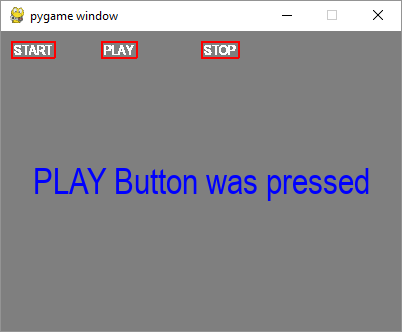# Pygame - Use Text as Buttons

Button is an important element in a typical game window. We can use a text or image surface object as button, so that when clicked it can fire a certain action.

Let us try to display three buttons with text captions.

text1=font.render(" START ", True, white)
text2=font.render(" PLAY ", True, white)
text3=font.render(" STOP ", True, white)


In order to draw a border around these buttons obtain their Rect object.

rect1 = text1.get_rect(topleft=(10,10))
rect2 = text2.get_rect(topleft= (100,10))
rect3 = text3.get_rect(topleft= (200,10))


Inside the event loop, blit the text buttons with red border around them.

screen.blit(text1, rect1)
pygame.draw.rect(screen, (255,0,0),rect1,2)
screen.blit(text2, rect2)
pygame.draw.rect(screen, (255,0,0),rect2,2)
pygame.draw.rect(screen, (255,0,0),rect3,2)
screen.blit(text3, rect3)


Use collidepoint() function of Rect object to identify which button has been clicked.

if event.type == pygame.MOUSEBUTTONDOWN:
if rect1.collidepoint(event.pos):
msg = "START Button was pressed"
if rect2.collidepoint(event.pos):
msg = "PLAY Button was pressed"
if rect3.collidepoint(event.pos):
msg = "STOP Button was pressed"


Display appropriate message as a text surface −

img=font.render(msg, True, (0,0,255))
imgrect=img.get_rect()
imgrect.center = (200 , 150 )
pygame.draw.rect(screen, bg, imgrect)
screen.blit(img, imgrect)


## Example

Following is the complete code −

import pygame

pygame.init()
screen = pygame.display.set_mode((400, 300))
done = False

font = pygame.font.SysFont("Arial", 14)
text1=font.render(" START ", True, white)
text2=font.render(" PLAY ", True, white)
text3=font.render(" STOP ", True, white)

rect1 = text1.get_rect(topleft=(10,10))
rect2 = text2.get_rect(topleft= (100,10))
rect3 = text3.get_rect(topleft= (200,10))
bg = (127,127,127)
msg=" "
screen = pygame.display.set_mode((400,300))
screen.fill(bg)
while not done:
for event in pygame.event.get():
screen.blit(text1, rect1)
pygame.draw.rect(screen, (255,0,0),rect1,2)
screen.blit(text2, rect2)
pygame.draw.rect(screen, (255,0,0),rect2,2)
pygame.draw.rect(screen, (255,0,0),rect3,2)
screen.blit(text3, rect3)

if event.type == pygame.QUIT:
done = True
if event.type == pygame.MOUSEBUTTONDOWN:
if rect1.collidepoint(event.pos):
msg = "START Button was pressed"
if rect2.collidepoint(event.pos):
msg = "PLAY Button was pressed"
if rect3.collidepoint(event.pos):
msg = "STOP Button was pressed"
img=font.render(msg, True, (0,0,255))
imgrect=img.get_rect()
imgrect.center = (200 , 150 )
pygame.draw.rect(screen, bg, imgrect)
screen.blit(img, imgrect)

pygame.display.update()


## Output

When each button is clicked, display window shows the following output −